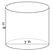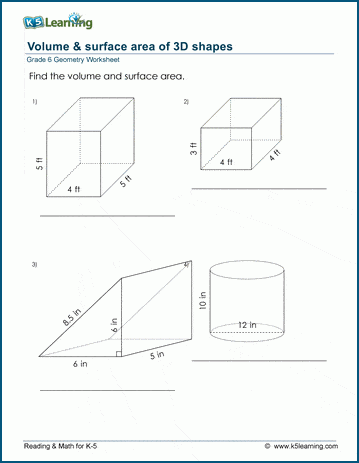# 6th Grade Math Worksheets: Geometry

## Geometry worksheets

These geometry worksheets give students practice in classifying shapes, finding perimeters, surface areas and volumes of 2-3 and 3-d shapes and other grade 6 geometry topics.

## Classify angles and shapes

Classify and measure angles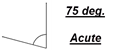Classify triangles by their sides
Equilateral, isosceles, scalene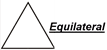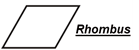## Area and perimeter

Area & perimeter of irregular rectangular shapes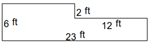Area of right triangles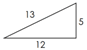Area of triangles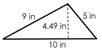Area of triangles, parallelograms and trapezoids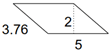## Circles

Circumference of a circle

Area of a circle

Circles: mixed practice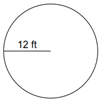## Rectangular prisms

Volume & surface area of rectangular prisms

Mixed practice with rectangular prisms
(with fractional lengths)

Mixed practice with rectangular prisms
(with decimals)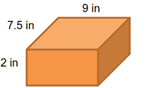Volume and surface area of 3D shapes